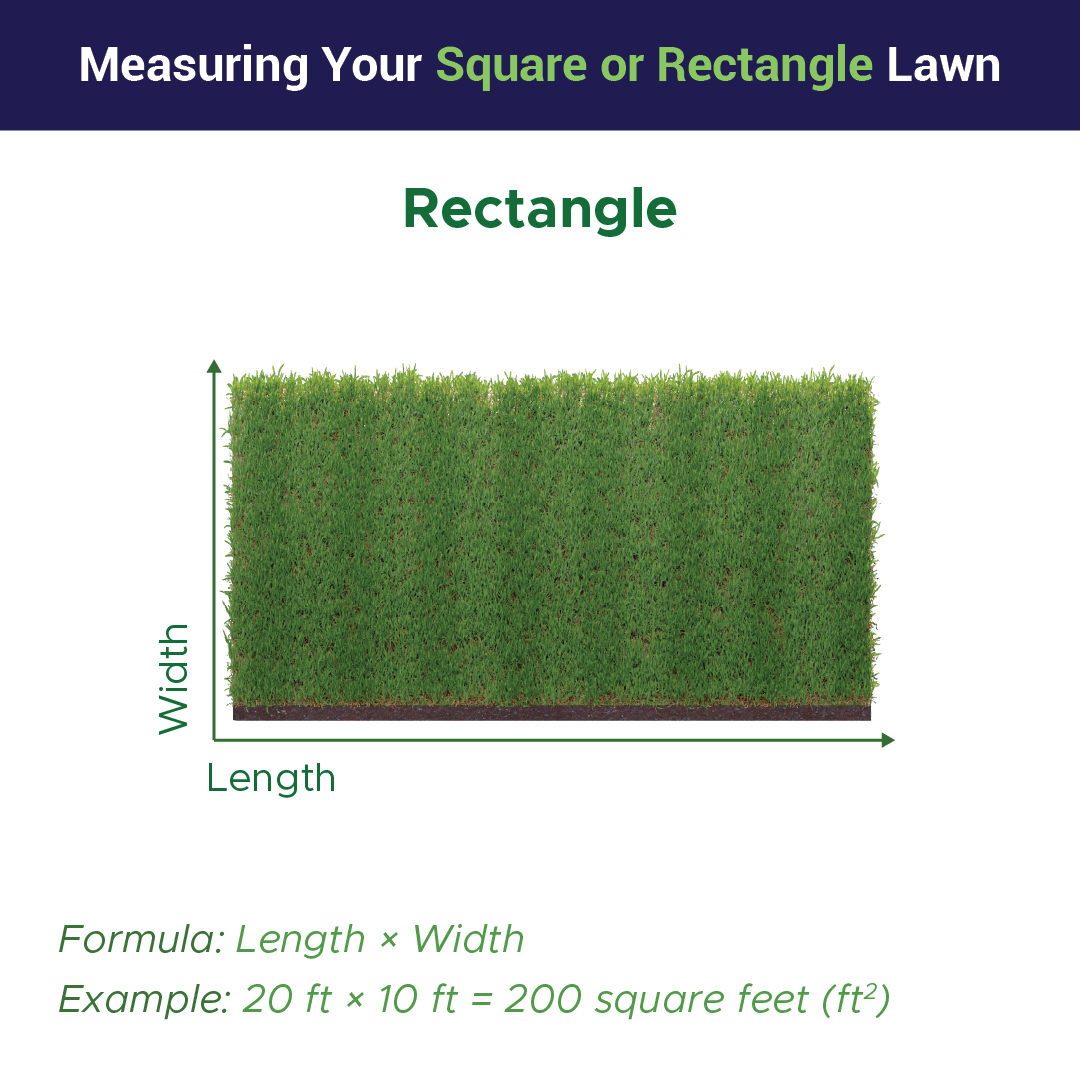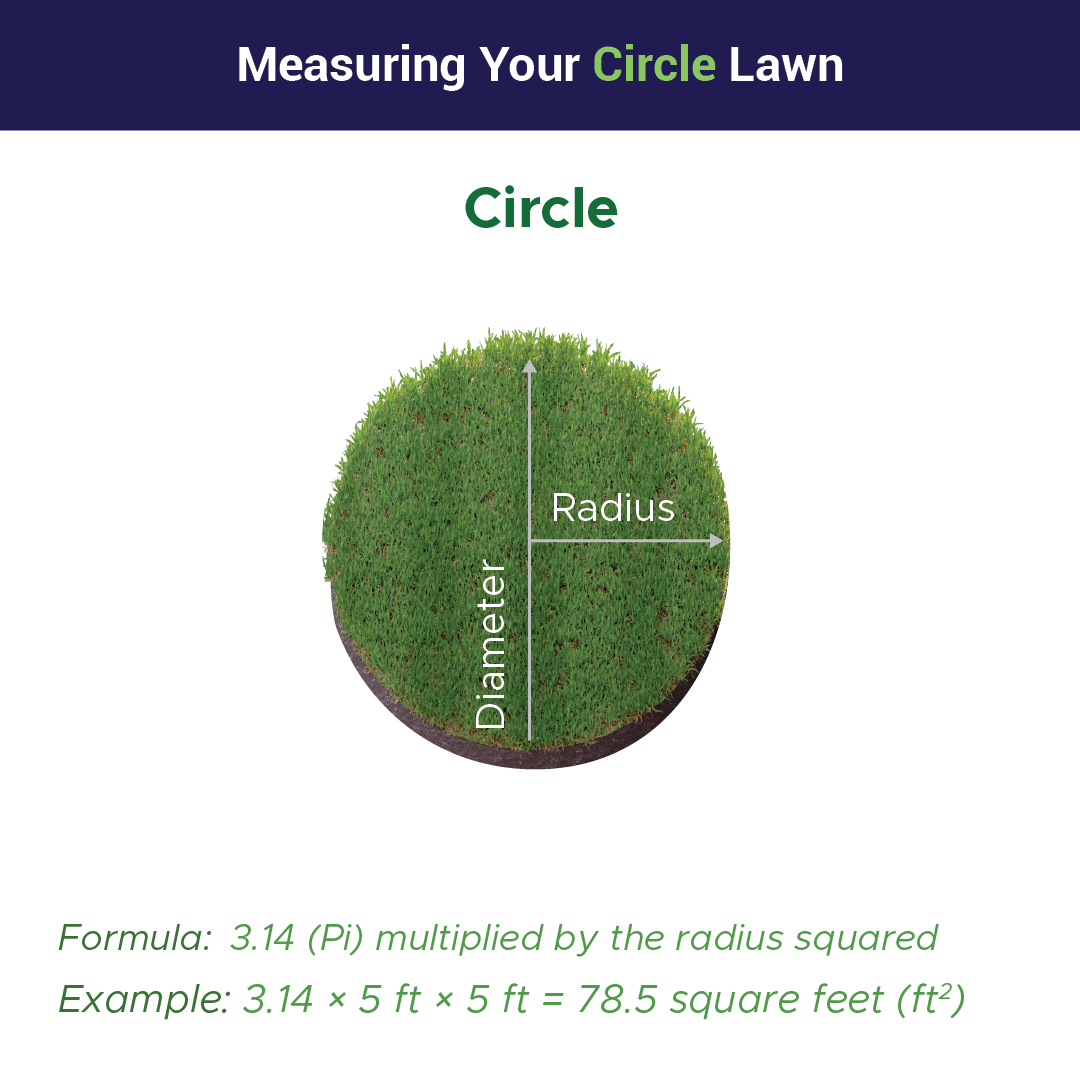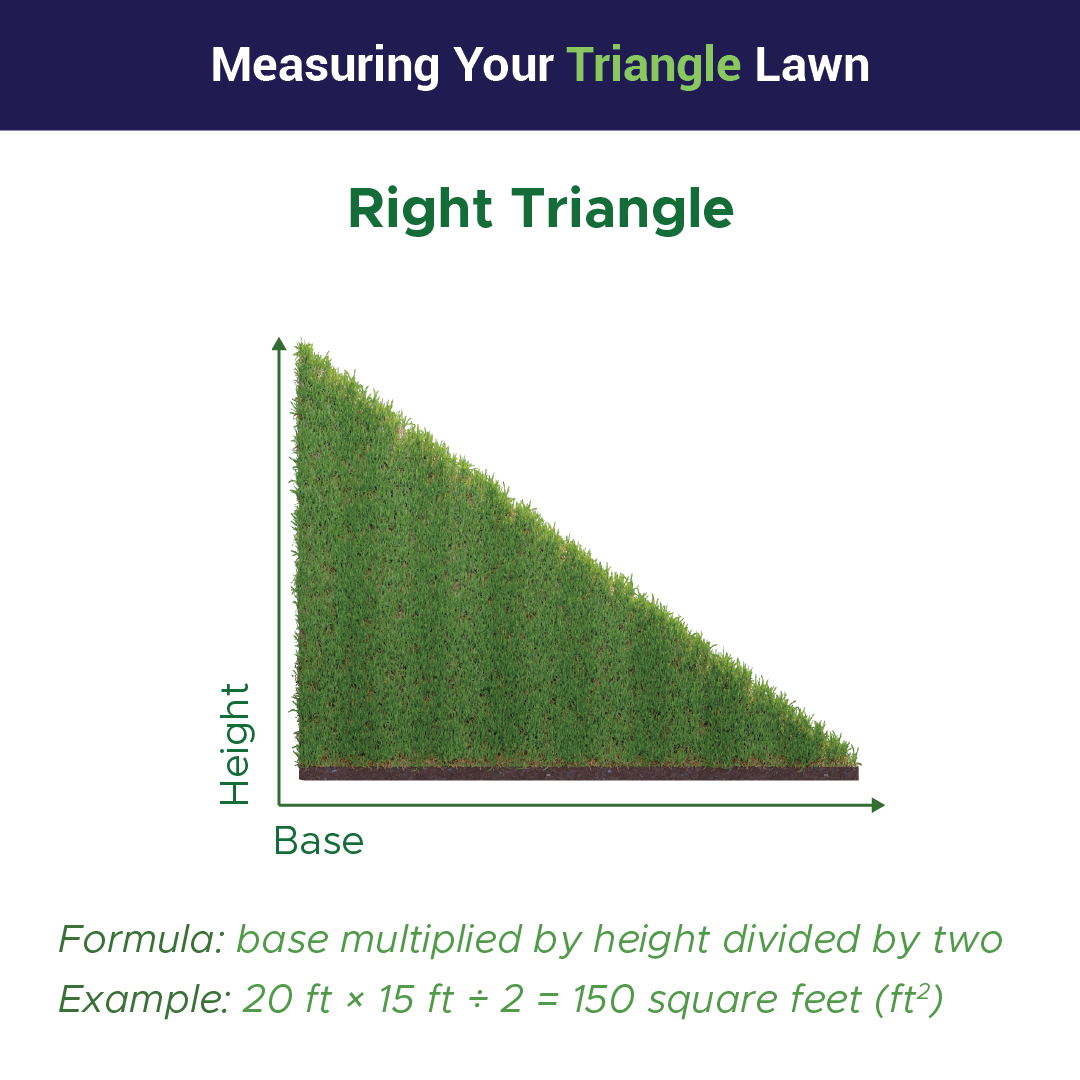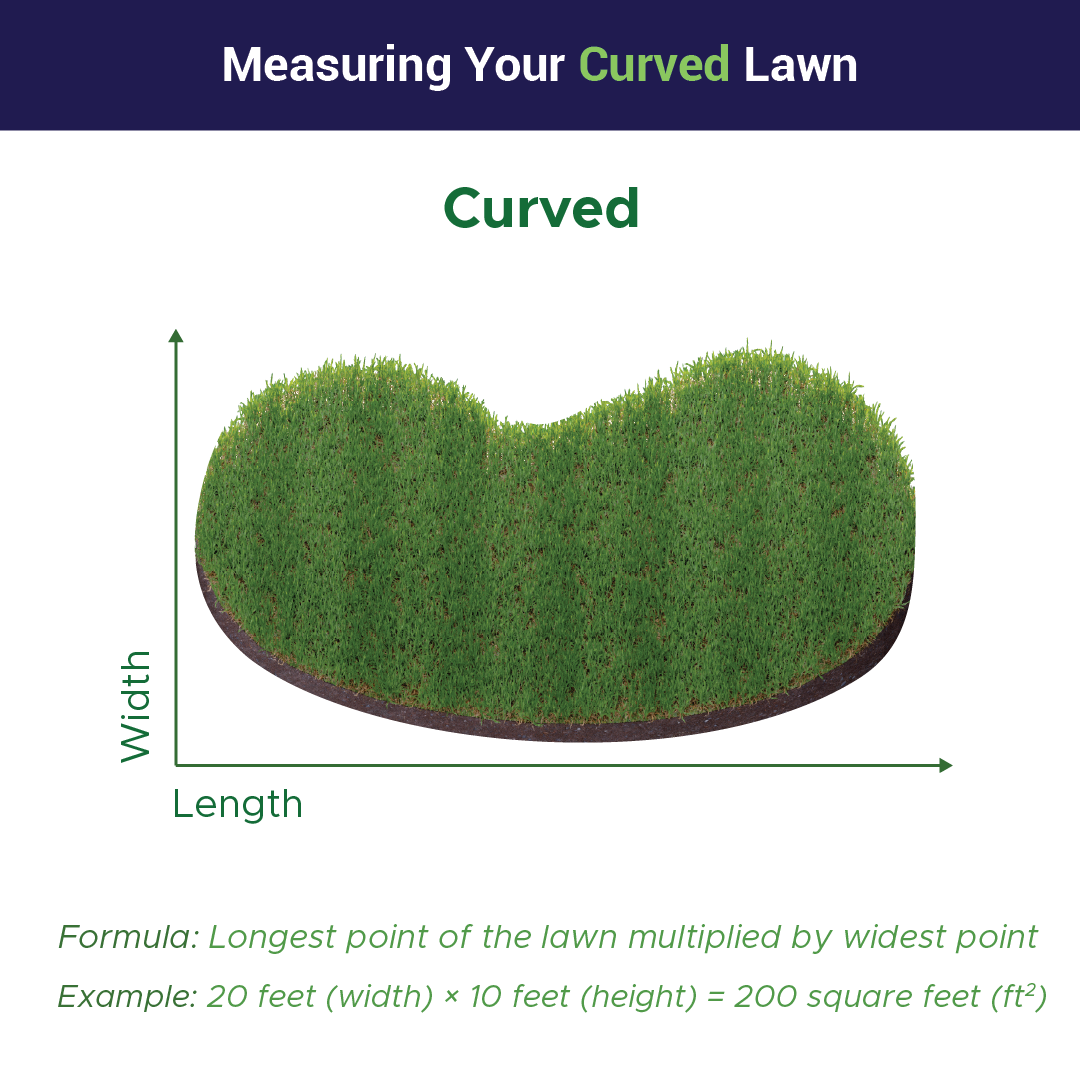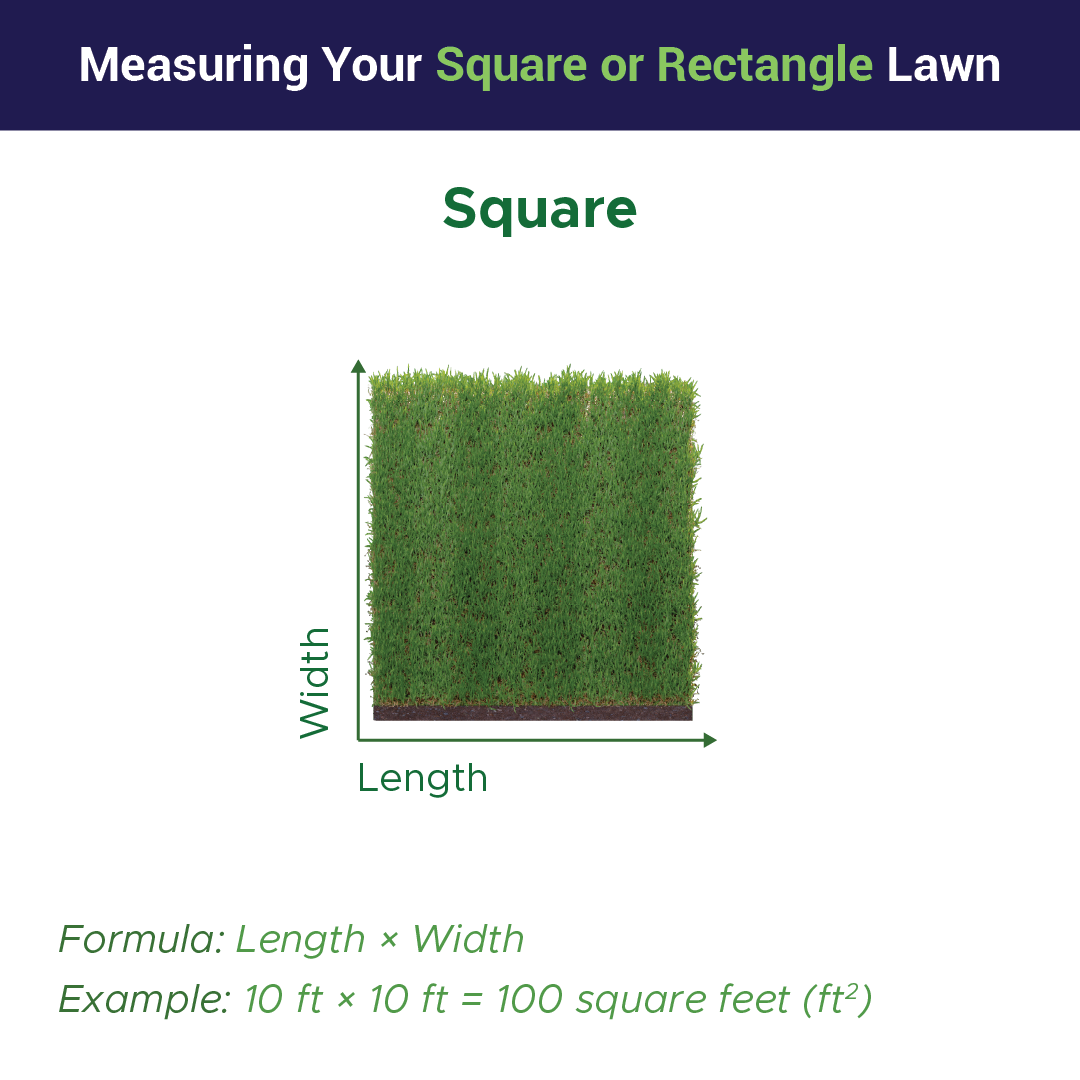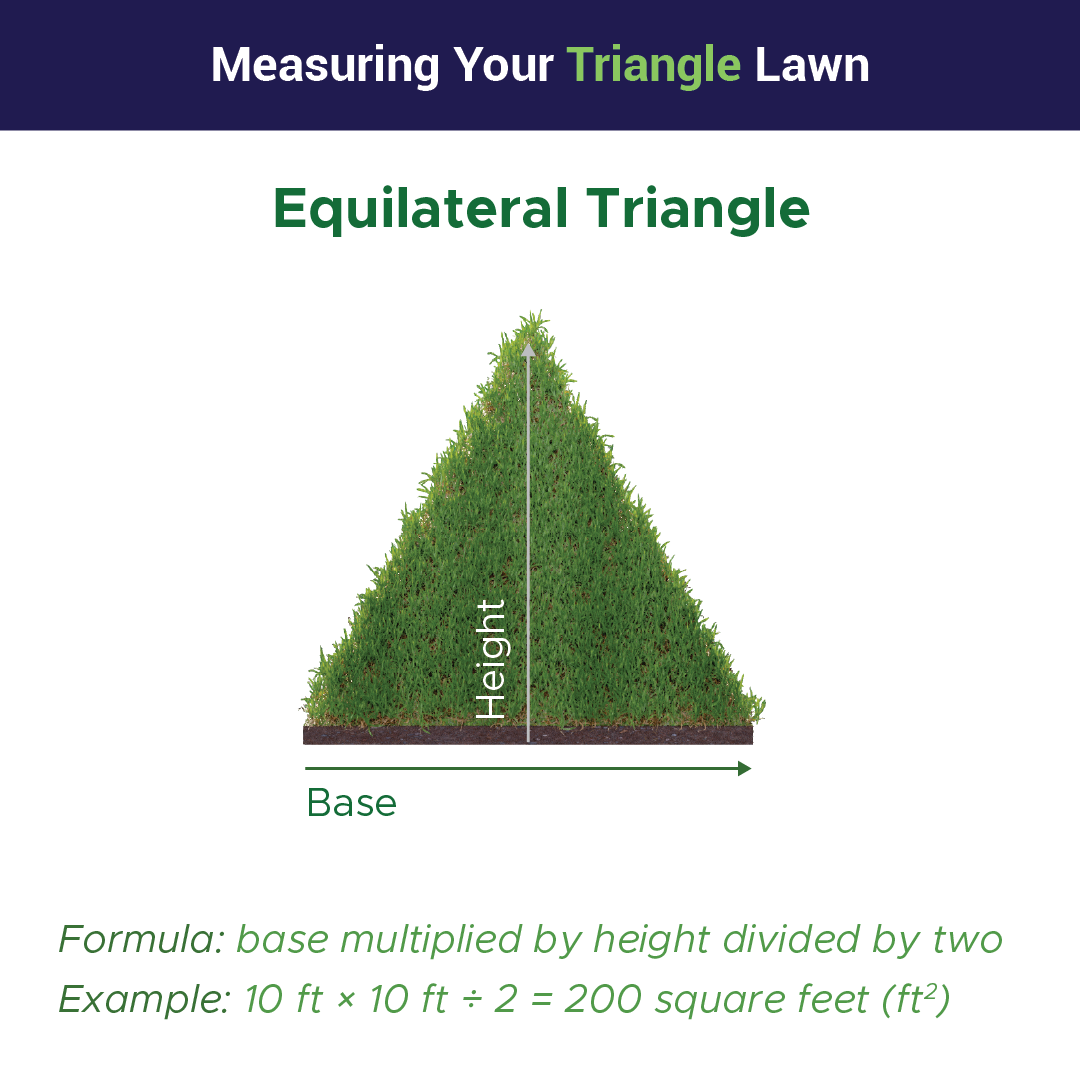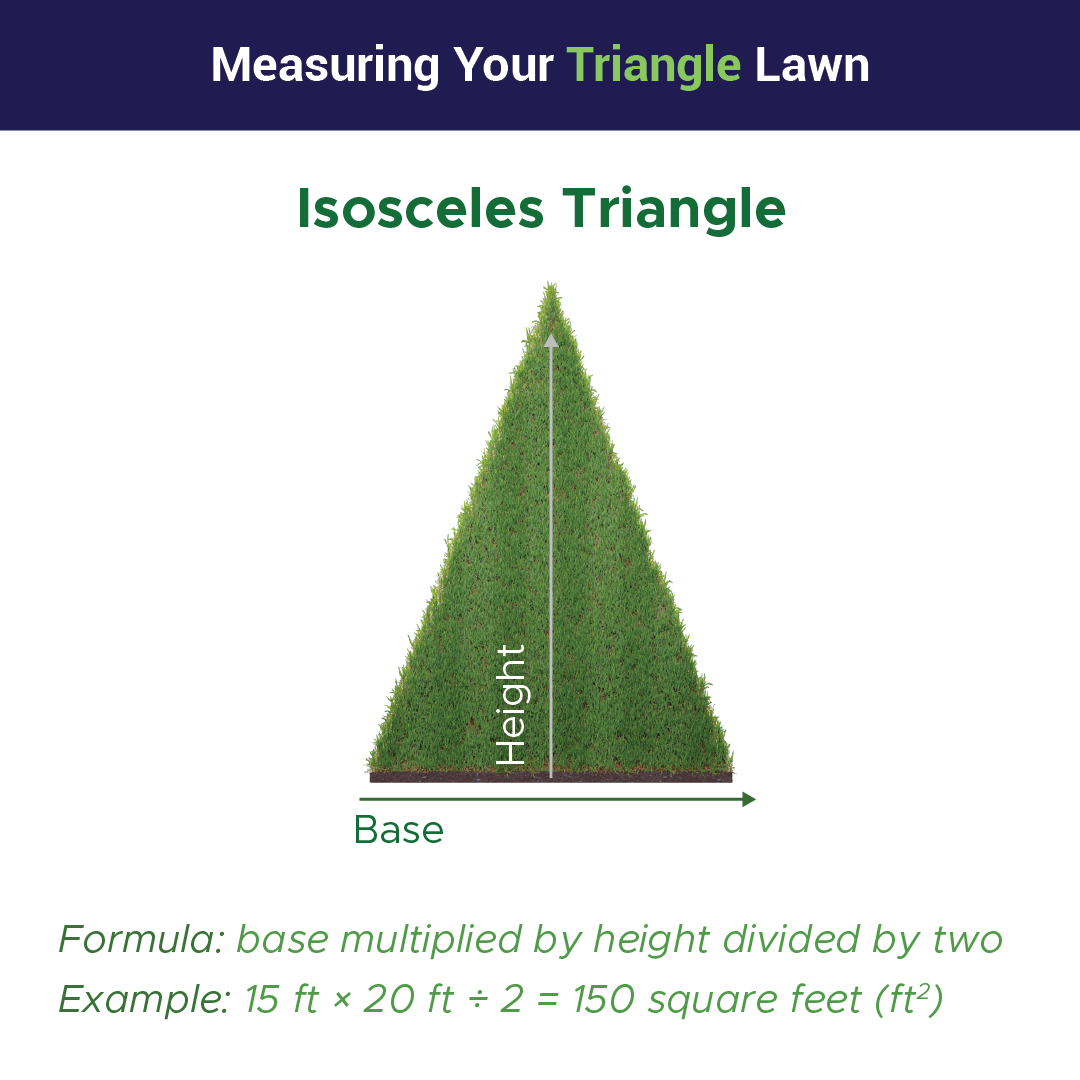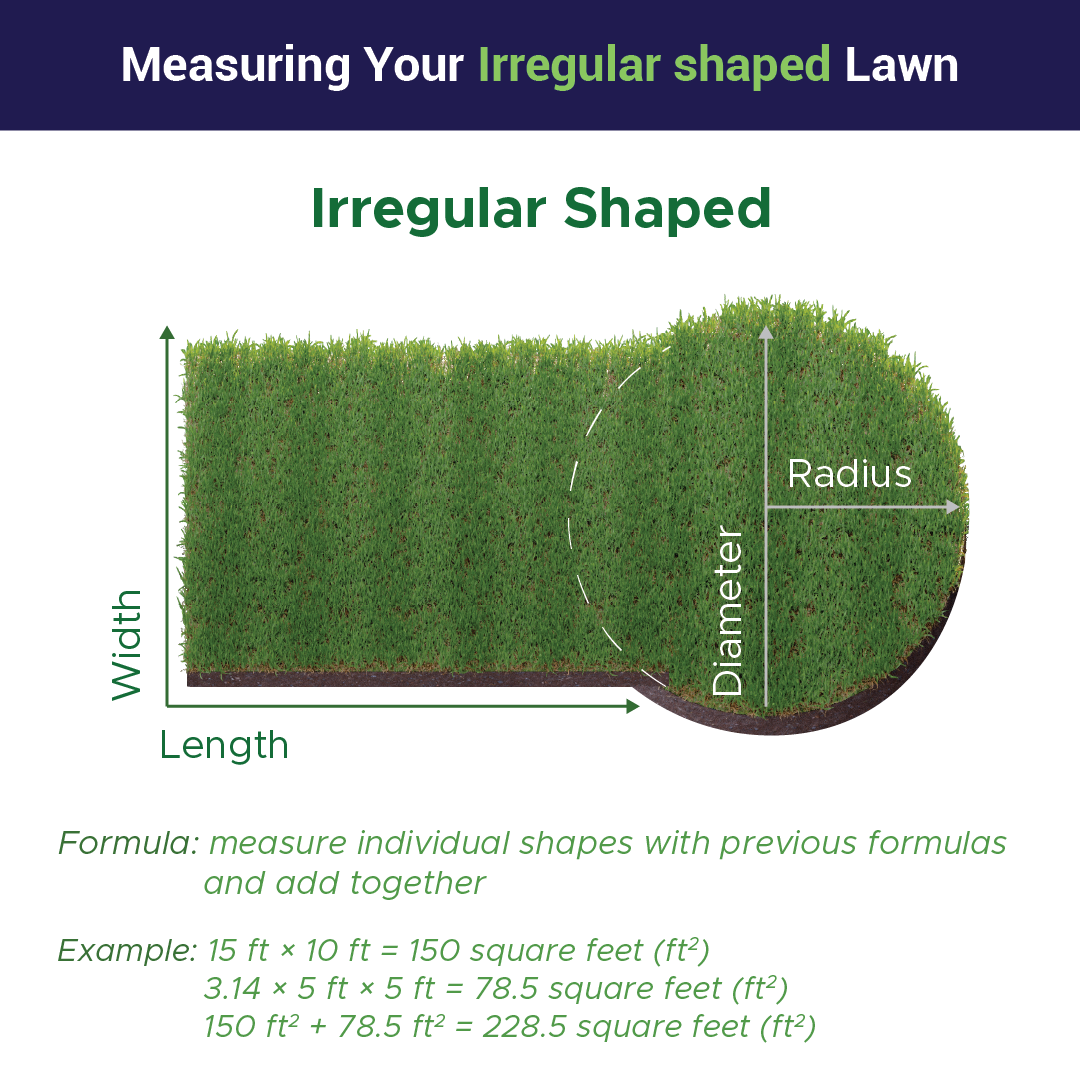In order to determine the most accurate amount of fertilizer to use, measure your lawn. Fertilizer application rates are typically displayed in units of pounds (lbs) per 1,000 square feet (ft2).

To measure, multiply the length of your lawn by its width. For lawns that have a more complicated shape, divide it into manageable squares, rectangles, triangles, and circles, then measure them and use the following formulas to calculate square footage. Total up all the measurements to find your lawn size.